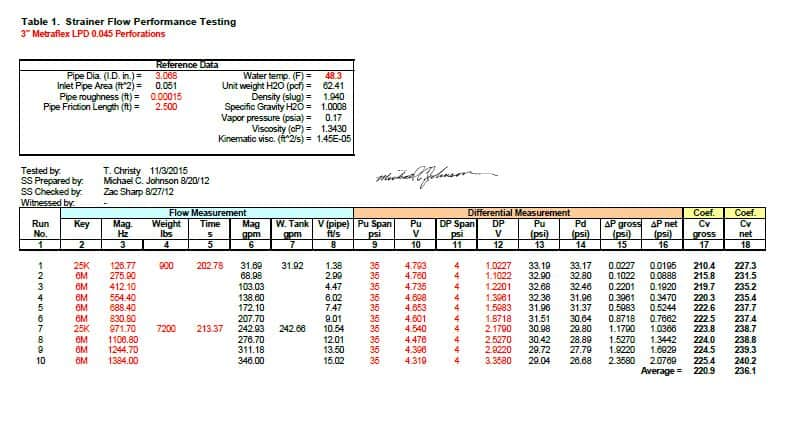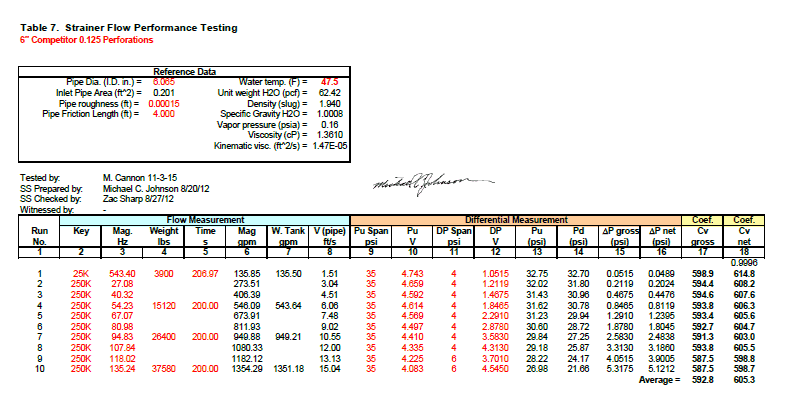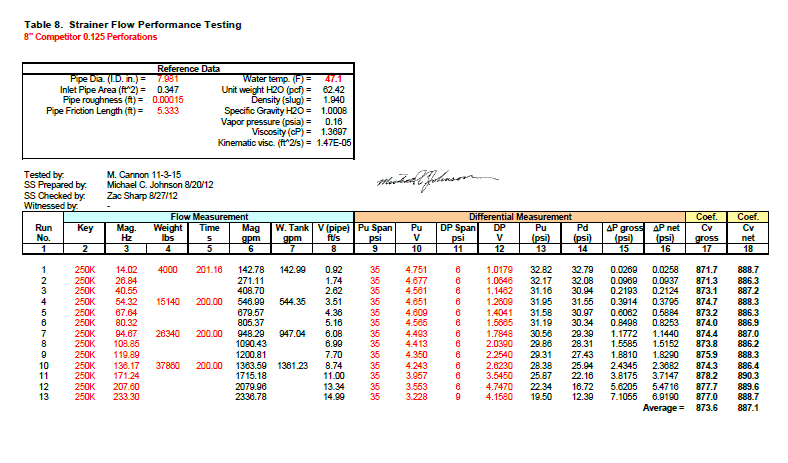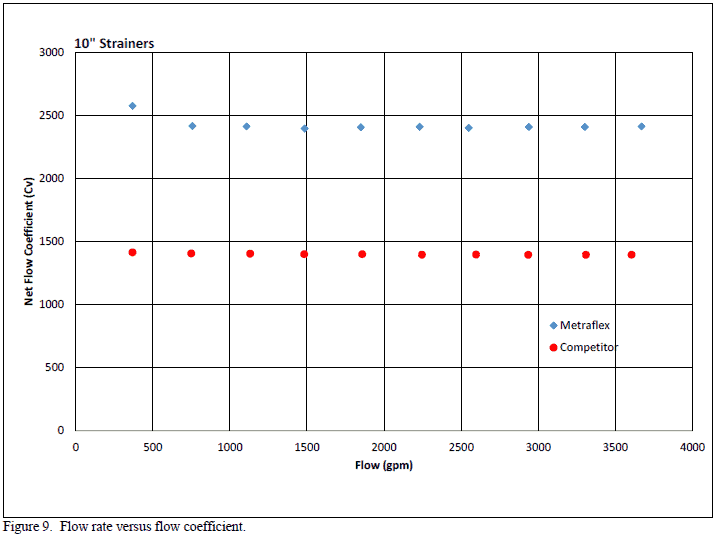Back

# LPD Y Strainer Flow Performance Testing at Utah Water Research Lab

#### Introduction

Flow performance tests were conducted at the Utah Water Research Laboratory (UWRL) on 3-inch, 4-inch, 6-inch, 8-inch and 10-inch Metraflex strainers. In addition, 4-inch, 6-inch, 8-inch and 10-inch Competitor strainers were also tested. The testing was developed to determine the flow versus pressure loss characteristics of each strainer and the flow coefficient of each strainer. Each strainer, with the exception of 3-inch Metraflex strainer and the 4-inch Competitor strainer, had strainer perforations measuring 0.125 inches in diameter. The 3-inch Metraflex strainer had 0.045 inch diameter perforations and the 4-inch Competitor strainer had 0.033 inch diameter perforations. The work was authorized under Metraflex Purchase Order No. 29582 and was done in accordance with the ANSI/ISA 75.02.01-2008 Control Valve Capacity Test Procedures standard with slight modifications in order to characterize each strainer’s performance over a wide flow range.

#### Experimental Program

Each of the strainers was installed in a test line (standard steel diameters) with approximately 20 diameters of straight approach piping to provide uniform flow at the inlet of the strainer. There were approximately 10 diameters of straight pipe downstream from the strainer. Pressure taps were located two pipe diameters upstream from the strainer and six pipe diameters downstream from the strainer. Flow was supplied with a 100 horsepower pump. Figures 1 through 4 show the test setup for the 4-inch and 10-inch strainers respectively. Each of the other sizes had similar installations however they were installed in pipe sizes corresponding to each strainer size.Figure 1. 4-inch strainer installation (Metraflex LPD Y-Strainer shown)Figure 2. 4-inch strainer installation (Competitor strainer shown).Figure 3. 10-inch strainer installation (Metraflex LPD Y-Strainer shown).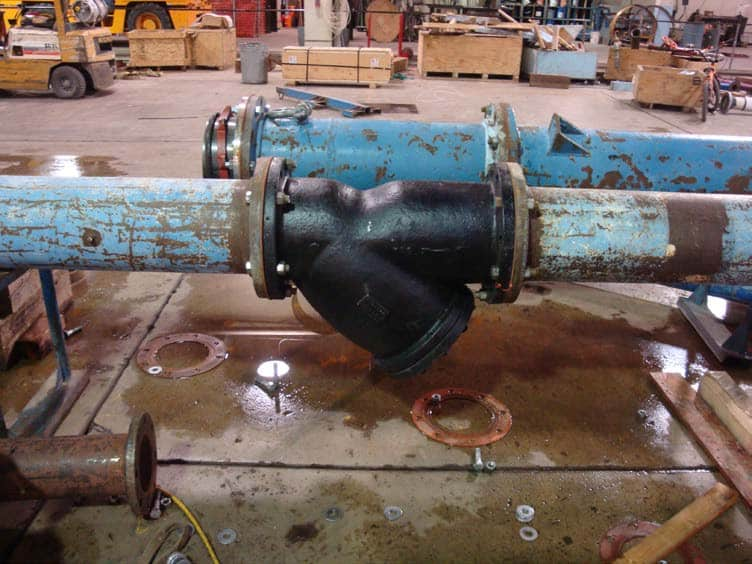Figure 2. 10-inch strainer installation (Competitor strainer shown).

The flow rate was measured using calibrated flow meters which were verified against certified weight tanks. The differential pressure across the strainer was measured using Rosemount differential transmitters. The upstream pressure was measured using a Rosemount transmitter. The water temperature was measured using a calibrated RTD.
Each strainer was tested over a range of flows sufficient to generate 1.5 ft/s to 15 ft/s average velocities in the approach pipe. Ten points were taken over the velocity range of each size.
In the case of the 6-inch Metraflex strainer, certain tests were completed to simulate strainer blockage. This was accomplished by using duct tape to completely block the screen in areas suspected to plug first. The plugged portion of the screen was side opposite the strainers inlet.
To prevent screen plugging during testing, Metraflex provided a 10-inch 20 mesh strainer that was used upstream to capture any debris that entered the test system from water supplied from Logan River

#### Flow Coefficient

The definition of the flow coefficient used in this report is: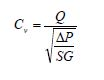Where Q is the discharge of test fluid in U.S. gallons per minute flowing through the strainer, ΔP is the pressure drop across the strainer in psi, and SG is the specific gravity of the test fluid. Cv is calculated using the gross pressure drop (ISA standard) between taps that are two diameters upstream and six diameters downstream.
The net flow coefficient was also computed by subtracting the friction expected from steel pipe between the gross differential pressure measured between the pressure taps. This calculation was completed using the Swamee-Jain equation to determine the friction factor associated with the specific data point taken in the laboratory. The Swamee-Jain equation is given by:Where f is the friction factor, k is the pipe roughness, D is the inside diameter of the pipe and Re is the Reynolds number of the flow in the pipe. The headloss in feet was then converted to psi and subtracted from the gross pressure loss measurement.

#### Test Procedure

The test procedure essentially followed ISA 75.02.01-2008 with slight modifications to account for the fact that a strainer is not a valve and it was desired to determine the strainer’s performance characteristics over a wide flow range.

#### Cv Determination

1. Install the strainer in straight piping of nominal size and standard wall thickness. Ensure that at least 20 diameters of straight pipe are upstream from the strainer and at least 8 diameters are installed downstream from the
2. Flow test the strainer at several different flow rates and observe the relationship between flow and Cv.
3. The following data shall be recorded:
1. Upstream pressure (measurement not to exceed 2 percent of actual value).
2. Pressure differential across the strainer (measurement not to exceed 2 percent of actual value).
3. Volumetric flow rate (measurement not to exceed 2 percent of actual value).
4. Fluid temperature (measurement error not to exceed 2 degrees Fahrenheit).
5. Strainer description and identifying
6. Calculate the gross and net flow coefficient Cv.

#### Test Results

The pressure loss and flow coefficient Cv data are given in Tables 1 through 9 and the net pressure loss and net flow coefficient data are shown graphically on Figures 5 through 14.